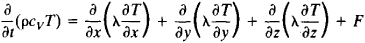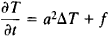Heat Equation

Also found in: Wikipedia.

heat equation

[′hēt i‚kwā·zhən]
(thermodynamics)
A parabolic second-order differential equation for the temperature of a substance in a region where no heat source exists: ∂ t /∂τ = (kc)(∂2 t /∂ x 2+ ∂2 t /∂ y 2+ ∂ t 2/∂ z 2), where x, y, and z are space coordinates, τ is the time, t (x,y,z, τ) is the temperature, k is the thermal conductivity of the body, ρ is its density, and c is its specific heat; this equation is fundamental to the study of heat flow in bodies. Also known as Fourier heat equation; heat flow equation.
McGraw-Hill Dictionary of Scientific & Technical Terms, 6E, Copyright © 2003 by The McGraw-Hill Companies, Inc.
The following article is from The Great Soviet Encyclopedia (1979). It might be outdated or ideologically biased.

Heat Equation

a parabolic partial differential equation that describes the process of propagation of heat in a continuous medium, such as a gas, liquid, or solid. It is the basic equation in the mathematical theory of thermal conductivity.

The heat equation expresses the heat balance for a small element of volume of the medium; heat gains from sources and heat losses through the surface of the element are taken into account for heat transport by conduction. The equation has the following form for an isotropic nonhomogeneous medium:Here, ρ is the density of the medium; cv is the specific heat of the medium at constant volume; t is time; x, y, and z are space coordinates; T = T(x, y, z, t) is the temperature, which is calculated by means of the heat equation; λ is the coefficient of thermal conductivity; and F = F(x, y, z, t) is the specified density of the heat sources. The magnitudes of ρ cv, and λ depend on the coordinates and, generally speaking, on the temperature. For an anisotropic medium, the heat equation contains in place of λ the thermal conductivity tensor λik, where i, k = 1,2,3.

In the case of an istropic homogeneous medium, the heat equation assumes the formwhere Δ is the Laplace operator, a2 = λ/(ρcv) is the coefficient of thermal diffusivity, and f = F/(ρcv). In a stationary state, where the temperature does not vary with time, the heat equation becomes the Poisson equation or, when there are no heat sources, Laplace’s equation ΔT = 0. The basic problems for the heat equation are the Cauchy problem and the mixed boundary value problem (seeBOUNDARY VALUE PROBLEMS).

The heat equation was first studied by J. Fourier in 1822 and S. Poisson in 1835. Important results in the study of the heat equation were obtained by I. G. Petrovskii, A. N. Tikhonov, and S. L. Sobolev.

REFERENCES

Carslaw, W. S. Teoriia teploprovodnosti. Moscow-Leningrad, 1947.
Vladimirov, V. S. Uravneniia matematicheskoi fiziki. Moscow, 1967.
Tikhonov, A. N., and A. A. Samarskii. Uravneniia matematicheskoi fiziki, 3rd ed. Moscow, 1966.

D. N. ZUBAREV

References in periodicals archive ?
On Space-Time Quasiconcave Solutions of the Heat Equation
In the present paper, we will apply GRPS to a series of PDE with variable coefficients, including fourth-order parabolic equations, fractional heat equation, and fractional wave equation.
We, firstly, utilise the heat equation [u.sub.t] - [u.sub.xx] = 0 as an illustrative example.
The diffusion equation is sometimes called a heat equation or a continuity equation and it plays an important role in a number of fields of science.
Using the notation [u.sup.n](x) = u(x, [t.sup.n]) and a similar format as Crank-Nicolson (C-N) scheme to approximate the heat equation (1) with second order at the time moment [t.sup.n+1/2] = ([t.sup.n+1] + [t.sup.n])/2, we have
Ismailov, "An inverse coefficient problem for the heat equation in the case of nonlocal boundary conditions," Journal of Mathematical Analysis and Applications, vol.
The first type is the heat equation with operator coefficient.
Therefore, it seems interesting to handle the mixed fractional heat equation. In the recent paper of Xia and Yan , they introduced only the existence and uniqueness of the solution of a mixed fractional heat equation driven by a fractional Brownian sheet.
It is based on the heat equation in a solid region where the phase change interface is regarded as a moving boundary (Stefan, 1891).
The impulsive effects on blow-up and quenching were first studied by Chan and Deng , Chan, Ke and Vatsala  for a semilinear heat equation, and Chan and Kong  for a degenerate semilinear equation.

Site: Follow: Share:
Open / Close